+

# Economies, Constant and Diseconomies of Scale

Author: Sophia Tutorial
##### Description:

Differentiate between economies, constant, and diseconomies of scale.

(more)### Developing Effective Teams

*No strings attached. This college course is 100% free and is worth 1 semester credit.

37 Sophia partners guarantee credit transfer.

299 Institutions have accepted or given pre-approval for credit transfer.

* The American Council on Education's College Credit Recommendation Service (ACE Credit®) has evaluated and recommended college credit for 32 of Sophia’s online courses. Many different colleges and universities consider ACE CREDIT recommendations in determining the applicability to their course and degree programs.

Tutorial
what's covered
This tutorial will cover how to recognize where a firm experiences economies, constant, and diseconomies of scale, based on how a firm's long run average is derived from its short run average cost curves.

Our discussion breaks down as follows:

1. Short Run vs. Long Run
1. Short Run
2. Long Run
2. LRAC and SRACs
3. Economies, Constant, and Diseconomies of Scale
4. Minimizing Average Costs in the Long Run
5. Economies of Scale: Example
6. Diseconomies of Scale: Example

## 1. Short Run vs. Long Run

Let's begin by discussing the difference between the long run and the short run.

Fixed and variable costs actually define this difference.

In the short run, there is at least one fixed input or cost. A firm cannot immediately expand their factory or get out of their current lease.

In the long run, all inputs or costs becomes variable. A firm can renegotiate their lease and make major changes to the firm.

Since we will be talking about average cost curves, here is a chart that calculates average total cost by taking the total cost column and dividing by the quantity.

Quantity Fixed Costs Variable Costs Total Cost Average Total Cost
0 \$100 \$0 \$100 --
1 \$100 \$30 \$130 \$130
2 \$100 \$50 \$150 \$75
3 \$100 \$60 \$160 \$53.30
4 \$100 \$72 \$172 \$43
5 \$100 \$85 \$185 \$37
6 \$100 \$110 \$210 \$35
7 \$100 \$140 \$240 \$34.30
8 \$100 \$180 \$280 \$35
9 \$100 \$230 \$330 \$36.70
10 \$100 \$290 \$390 \$39

Notice how the average total cost falls at first, then eventually rises.

Average cost curves will be this shape, which you will see graphically, but this represents just one short run scenario.

1a. Short Run

In the short run, a firm will be operating on a fixed scale, because there is at least one fixed input.

Now, they can certainly vary some things, like the number of workers they hire or machines they purchase, but there is at least one input that is fixed, like the size of their factory.

As shown above in the chart, where average cost is falling at first and then rising, this results in a u-shaped short run average cost curve.

Average costs fall at first because of economies of scale and factors such as specialization of the workers, but they eventually rise because of the law of diminishing marginal returns.

The law of diminishing returns shows that at some point when hiring workers, while that next worker hired will certainly add to total production, he or she won't add as much in terms of production as the worker before, because they are operating on a fixed scale.

In the short run, given the constraints faced, the firm will maximize profit or minimize loss.

1b. Long Run

In the long run, which is the subject of this tutorial, all inputs and costs become variable.

Now the firm can alter its factory size and therefore really affect their scale of operation, which simply refers to how big they are going to get.

EXAMPLE

For example, a local business might decide to open up more locations across a region and operate on a much bigger scale instead of just having one retail outlet, which would only be possible for a business in the long run.

The long run average cost curve will be u-shaped. Economies of scale are going to be where average costs fall as the company decides to grow in size, while diseconomies of scale will be seen when average costs rise as the company gets bigger, or increase the scale of production.

## 2. LRAC and SRACs

Here is what the long run average cost (LRAC) curve looks like.

The long run average cost curve is made up of each of these short run average cost (SRAC) curves. There are seven SRACs shown here, and each one represents a different short run scenario.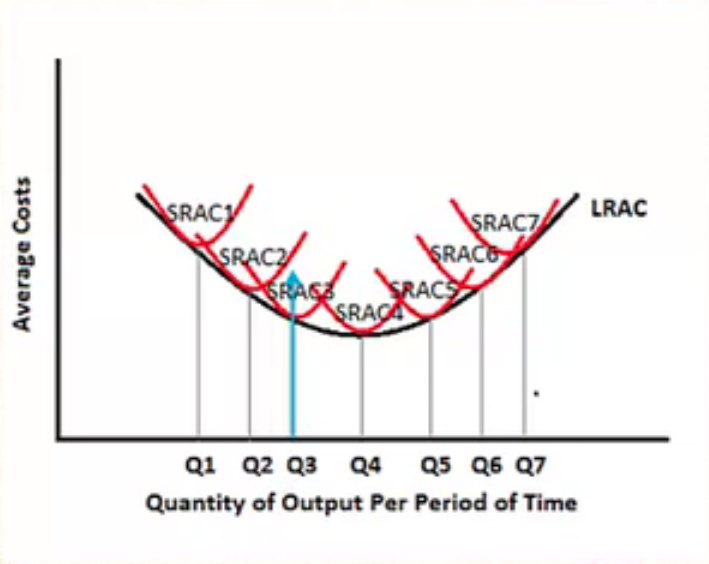Short run average cost curve one would be the smallest scale of operation, while seven would be the largest scale of operation on this graph.

We take the lowest points of each of these short average cost curves to derive our long run average cost curve.

Now, if a firm wanted to produce at output level quantity 3, for instance, they would see which SRAC scale would minimize their costs.

As you can see, if they want to produce this quantity, average cost curve 2 that would place them too far up the curve in terms of average cost. So, scale 2 would be too small of a scale.

However, they're not big enough to justify moving over to short run average cost curve 4.

Short run average cost scenario 3 would be best choice, if they wanted to produce quantity 3.

## 3. Economies, Constant, and Diseconomies of Scale

Again, the long run average cost curve will always be equal to or lie below the short run average cost curves.

This is because a firm will never produce at a higher average cost in the long run versus the short run, because all inputs are variable and there are no constraints to minimizing cost.

Let's remove the short run average cost curves from the graph so we can focus on the long run average cost.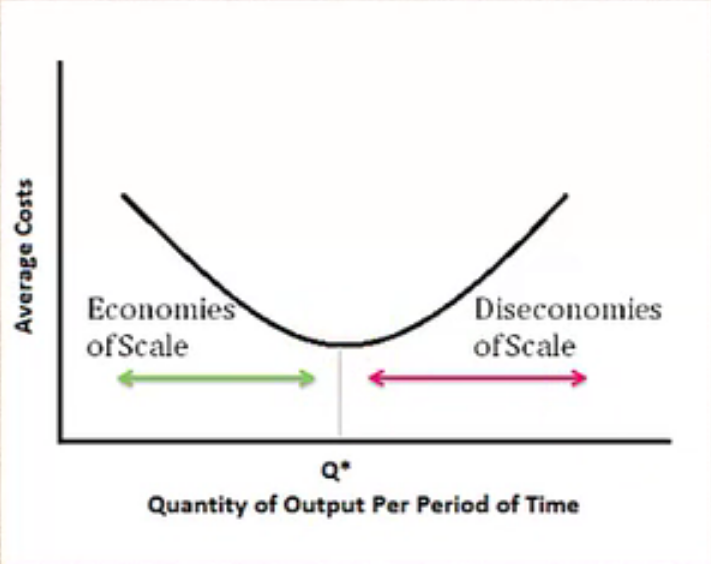Up to point Q*, notice how the long run average cost curve is falling. This is where the firm is experiencing increasing returns to scale, or benefits from increasing their scale of production.

This is economies of scale, which is an increase of operational facilities that create a decrease in marginal cost, or sometimes you will see this as average cost.

They are benefiting from expanding or growing in size, as you can see on the x-axis.

However, beyond point Q*, average costs begin to rise, so this would be an increase in cost region. The firm experiences decreasing returns to scale, meaning they are not getting a lot out of growing in size.

This is not economical, so it is diseconomies of scale, which is an increase of operational facilities that create an increase in marginal cost.

There are diminishing benefits here from increasing their scale of production.

Where the long run average cost curve might be flat on this graph--a small region around point Q*--would represent constant returns to scale. There is no change in average costs from increasing scale of production.

Therefore, in this small region, it would be constant returns.

terms to know
Economies of Scale
An increase of operational facilities that create a decrease in marginal cost
Diseconomies of Scale
An increase of operational facilities that create an increase in marginal cost

## 4. Minimizing Average Costs in the Long Run

Ultimately, the goal is to find which scale of operation will minimize average costs in the long run.

In the long run, there aren't constraints, so the firm can adjust to changing market conditions.

According to this graph, the quantity and scale of operation identified by the arrow would minimize their average cost in the long run.

This firm will experience economies of scale up to SRAC4 and diseconomies of scale beyond that point.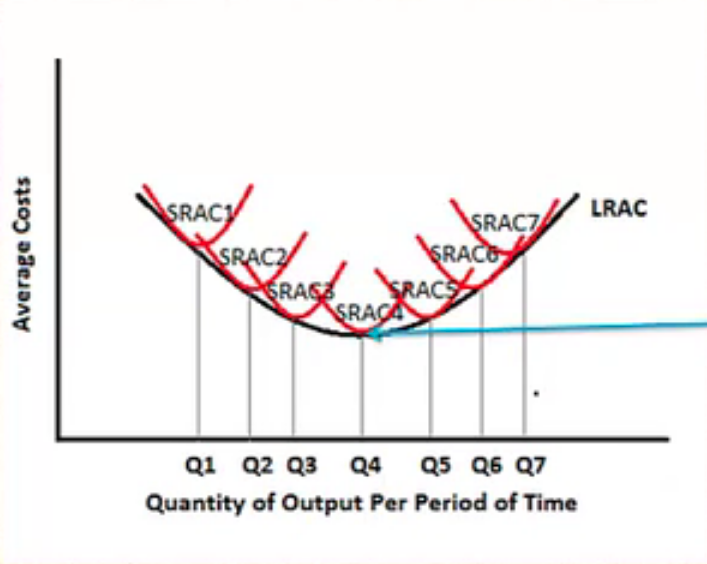## 5. Economies of Scale: Example

Let's explore an example of economies of scale.

Notice that it has been drawn so that average costs are never increasing.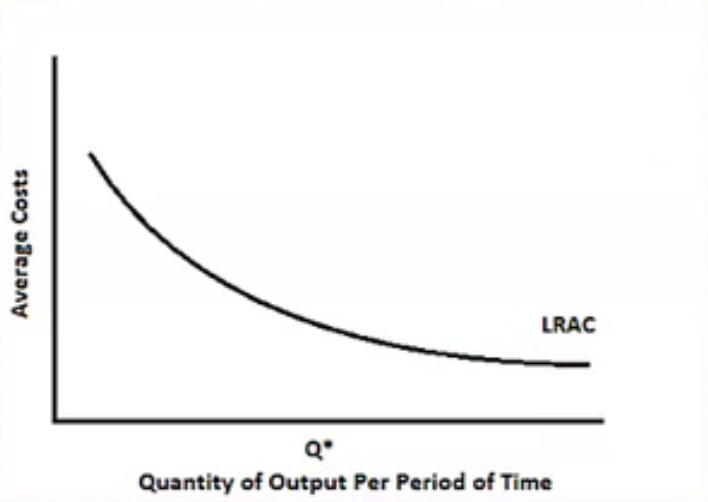You will sometimes see this as an example with utility companies, who generally experience economies of scale.

If you think about it, utility companies have large upfront costs that they need to cover.

They have to put an entire network in place, depending on what kind of utility company they are, and that cost is extreme.

Once that network is in place, the additional cost of servicing another home is fairly low as long as the network is in that area, and because of this, as they service more and more homes, their average costs fall, because they can spread out those large upfront costs.

This is why many utility companies are referred to as natural monopolies, meaning it is standard for them to grow in size because of the cost advantage that they gain.

## 6. Diseconomies of Scale: Example

Now, looking at an example of diseconomies of scale, it is possible for a firm to get too large. It can be quite inefficient once a company grows too big.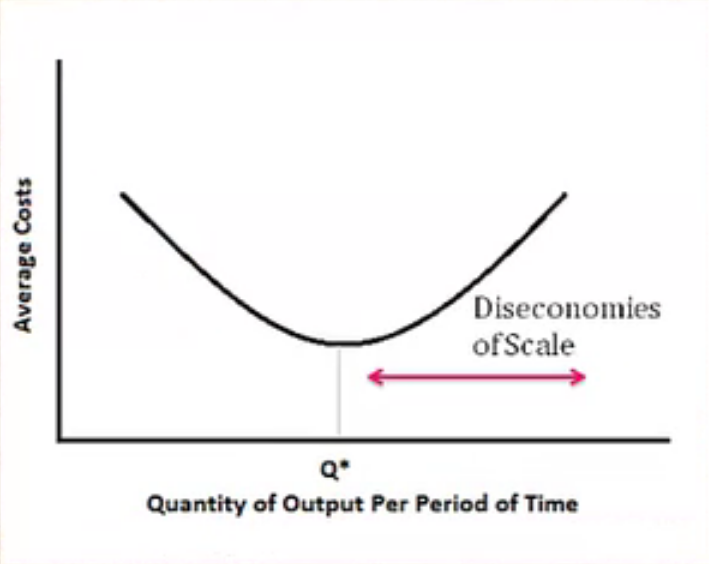Inefficiencies that can result with overly grown companies include:

• Poor communication
• Coordination problems between departments and divisions of the company
• Employees, detached from the company and owners, making decisions that are not in the best interest of owners

Therefore, it can become inefficient and average costs begin to rise.

summary
We began today's lesson by discussing the difference between the short run vs. long run, a difference defined by fixed and variable costs. We learned that a firm's long run average cost (LRAC) curve is derived from its short run average cost (SRAC) curves, each representing a different scale of production. We learned that where average costs fall as scale increases, the firm experiences economies of scale, while where average costs rise as scale increases, the firm experiences diseconomies of scale. Where there is no change in average costs from increasing scale of production, this represents constant returns.

We learned that ultimately, the goal is to find which scale of operation will minimize average costs in the long run. Finally, we wrapped up the tutorial by exploring examples of economies and diseconomies of scale.

Source: Adapted from Sophia instructor Kate Eskra.

Terms to Know
Diseconomies of Scale

An increase of operational facilities that create an increase in marginal cost.

Economies of Scale

An increase of operational facilities that create a decrease in marginal cost.

Rating